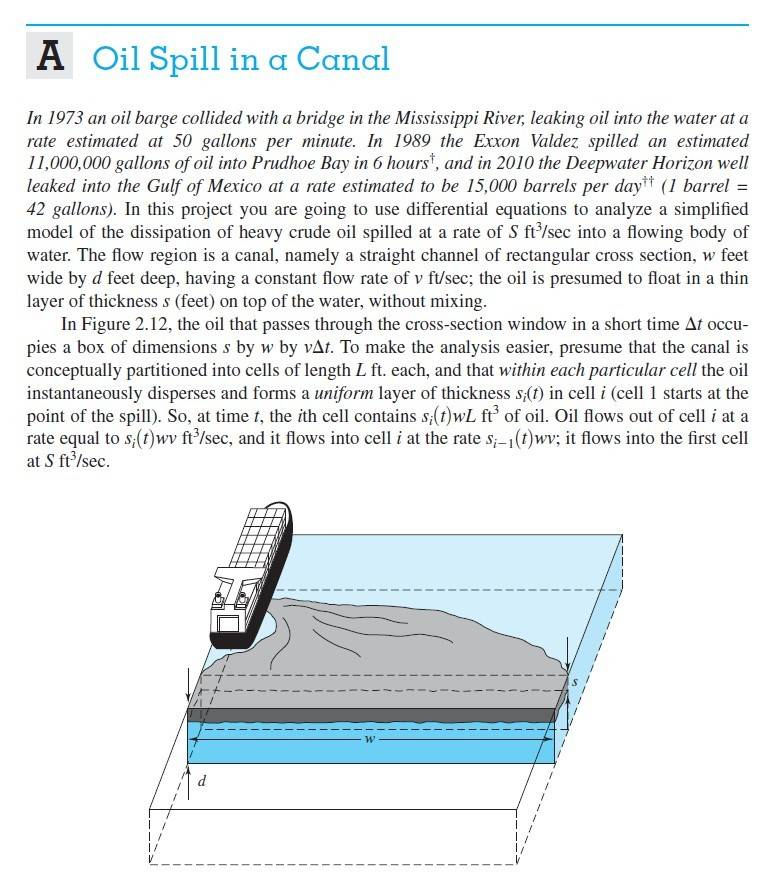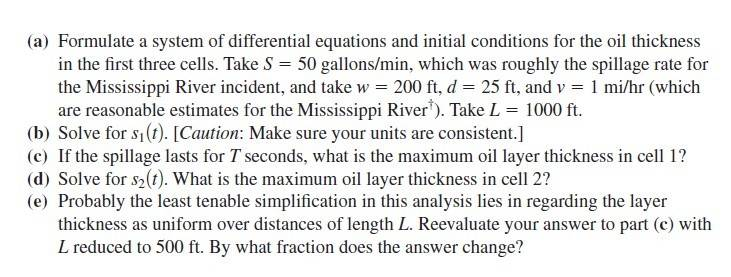# Oil Spill - Differential Equations

• MHB
• echianne
In summary, the conversation is about a problem with understanding a system of differential equations involving oil flow in cells. The volunteer suggests that more information is needed and asks what the person has tried. The crucial information is then provided, explaining the rate of oil flow between cells and the derivative of the amount of oil in each cell.

#### echianne

Hello everyone. I hope anyone can help me with this problem. I will greatly appreciate it. Willing to compensate anybody to answer this problem correctly for me.echianne said:
Hello everyone. I hope anyone can help me with this problem. I will greatly appreciate it. Willing to compensate anybody to answer this problem correctly for me. View attachment 11832View attachment 11833
This is a free forum. We volunteer our time... payment is not necessary. However in order to help you best we need to have an idea of just where you are running into problems. What are you able to do with this?

-Dan

topsquark said:
This is a free forum. We volunteer our time... payment is not necessary. However in order to help you best we need to have an idea of just where you are running into problems. What are you able to do with this?

-Dan
Thank you so much. I have come up with different systems of DE but nothing seemed to work. I think there is something wrong with how I understood the problem.

echianne said:
Thank you so much. I have come up with different systems of DE but nothing seemed to work. I think there is something wrong with how I understood the problem.
What have you tried?

-Dan

The crucial information is in the last two sentences: "So at time t, the ith cell contains $s_i$ wL $ft^3$ of oil. Oil flows out of cell i at a rate equal to $s_i(t)$ wv $ft^3/s$ and flows into cell i at a rate equal to $s_{i-1}(t)$ wv $ft^3/s$. It flows into the first cell at rate S $ft^3/s$".

That is just saying that the rate oil flows into one cell is the rate at which it flows out of the previous cell.
The DERIVATIVE of the amount of oil ($s_i(t)$) at a given x (at a given cell) is the rate at which oil is flowing in minus the rate at which it is flowing out: $\Delta s_i(t)= (s_{i-1}(t)- s_i(t))\Delta t$.
$\frac{\Delta s_i(t)}{\Delata t}= (s_{i-1}(t)- s_i(t))= -(s_i(t)- s_{i-1})$.

Taking the limit as the length of each of each cell goes to 0, $\frac{ds}{dt}= s$ with initial value s(0)= S.